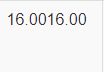# Adding the values of column together to display to another column

``````                {
"data": null,
"render": function(data,type,row) { return (data["valueA"] + data["valueB"])} //Try to add these value
},
``````

When trying to use the "+" symbol, nothing happens and only show the valuesA and valueB like this (16.0016.00)
When using the (* and -) symbol, it works as intended. Was wondering if there was a way to add them together

• symbol

Not sure what you mean.

Looks like `data["valueA"]` and `data["valueB"]` are being treated as stirngs. You can convert them. Try this:

`return ( Number(data["valueA"] ) + Number(data["valueB"]) )`

Kevin

• I've tried the suggestion above. The ouput comes out as NaN .
In terms of symbol, this is what I mean. As seen in the screenshots below (Both valueA & valueB are 16). When trying to do minus & multiply, they output both as 256 & 0. But when trying to add them together, it just output as "16.0016.00"

``````                {
"data": null,
"render": function(data,type,row) { return (data["valueA"] + data["valueB"])}
},
``````Minus -

``````                {
"data": null,
"render": function(data,type,row) { return (data["valueA"] + data["valueB"])}
},
``````Multiply *

``````                {
"data": null,
"render": function(data,type,row) { return (data["valueA"] + data["valueB"])}
},
``````• edited September 19

16.0016.00
That is string concatenation.

I don't understand your examples. The code is the same in each of them.

If you are sure the data type is string, try parseInt().
https://www.w3schools.com/jsref/jsref_parseInt.asp

• ``````            columns: [
{
data:'valueA',
},
{
data:'valueB',
},
{
"data": null,
"render": function(data,type,row) { return (data["valueA"] + data["valueB"])}
},
{
defaultContent: '<input type="button" class="Edit" value="Edit"/><input type="button" class="Delete" value="Delete"/>'
},

]

``````

I've made a mistake in the above comment as the opeartors are not correct.
This is the code for the table, trying to get the sum of the 2 columns and output it in another column

• This example shows what tangerine and I are talking about:
http://live.datatables.net/hekubina/1/edit

Both a and b are strings. Multiplying them works but adding them doesn't unless you convert them.

If you need help with this then please post a link to your page or a test case so we can help debug. Otherwise you can use `console.log` and `typeof` to debug the data types for `data["valueA"` and `data["valueB"`. Or use the browser's debugger.

Kevin

• I've tried the suggestion above. The ouput comes out as NaN .

What was your return statement when you got this result?

Kevin

• ``````                {
"data": null,
"render": function(data,type,row) { return (Number["valueA"] + Number["valueB"])}
},
``````

This is the return statement. Its kinda difficult to show the program as it is reading from a DB, so you wouldn't be able to see the full program. Below is something similar to what I want, but, Value A & B are coming from a DB table. Is there a way to change the data type to number?

``````|Value A    |Value B    |Value C
|     3     |     5     |     8     |
|     4     |     2     |     6     |
|     5     |     3     |     8     |
``````
``````return parseFloat(data['valueA']) + parseFloat(data['valueB']);
• Yep that did the trick, thanks allan